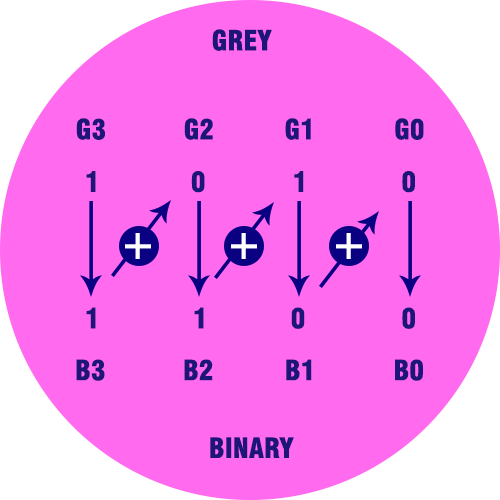﻿ Gray to Binary Code Converter Calculator | Calculators4U

# Gray to Binary Code Converter

Use our gray to binary code converter is a free online calculator / conversion tool to convert gray code value into binary number format.

## Gray Codes

Gray Code is a form of binary that uses a different method of incrementing from one number to the next. With Gray Code, only one bit changes state from one position to another.

## Binary Codes

A symbolic representation of data / information is called code. The base or radix of the binary number is 2. Hence, it has two independent symbols. The symbols used are 0 and 1. A binary digit is called as a bit. A binary number consists of sequence of bits, each of which is either a 0 or 1. Each bit carries a weight based on its position relative to the binary point. The weight of each bit position is one power of 2 greater than the weight of the position to its immediate right. e. g. of binary number is 101011 which is equivalent to decimal number 43.### Converting Gray Code to Natural Binary

On paper, the process is easy:

1. Write the Gray Code and copy the left most bit under itself.
2. Add the copied bit to the next Gray Code bit to the right, drop all carries, and write result next to the copied digit from step 1. Remember, 0+0=0, 0+1=1, 1+0=1, 1+1=0.
3. Repeat step 2 until completed.

### Applications of Gray Code

In instrumentation and data acquisition systems, where linear or angular displacement is measured. In shaft encoders, input-output devices, A/D converters and the other peripheral equipment

### Binary to Gray Code Truth Table:

Decimal Binary Code Gray Code
0 0000 0000
1 0001 0001
2 0010 0011
3 0011 0010
4 0100 0110
5 0101 0111
6 0110 0101
7 0111 0100
8 1000 1100
9 1001 1101
10 1010 1111
11 1011 1110
12 1100 1010
13 1101 1011
14 1110 1001
15 1111 1000